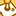# New to Qlik Sense

If you’re new to Qlik Sense, start with this Discussion Board and get up-to-speed quickly.

cancel
Showing results for
Did you mean:Contributor III

## Convert SQL functionality to Qlik functionality

I would like to convert three subqueries in SQL to the equivalent QLIK commands. Essentially to reduce the extra charge on the DB2 database

The first query is the first entry of any ticket so we need this to calculate how long the ticket has been open
ROW_NUMBER is 1 this will be the first ticket

WITH DMD_FR AS
(SELECT
DEMANDE_ID
,DEMANDE_CREATION
,ROW_NUMBER() OVER (PARTITION BY DEMANDE_ID ORDER BY DEMANDE_DATE || DEMANDE_TIME DESC) AS ROW_NUMBER
FROM
\$(vServer).DEMANDE
WHERE
STATUT='02'
AND DEMANDE_CREATION >= '\$(vOLDOPEN)'),

The second query is the latest entry of any unclosed ticket
DMD_LTST AS
(SELECT
DEMANDE_ID
,MAX(DEMANDE_DATE || DEMANDE_TIME) AS DEMANDE_LTST
FROM
\$(vServer).DEMANDE DMD_LTST
WHERE
STATUT IN ('02', '06', '07')
AND IDRC = '3340635'
AND DEMANDE_CREATION >= '\$(vOLDOPEN)'
AND DEMANDE_MODIF_CODE IN ('0000', '0023', '0024', '8803')
AND NOT EXISTS (SELECT 1 FROM
DB2PROD.DEMANDE DMD_88
WHERE DMD_88.DEMANDE_ID = DMD_LTST.DEMANDE_ID
AND DMD_88.STATUT = '88' )
GROUP BY DEMANDE_ID )

This is the main query, so I am assuming that if I load this first, I could then run two QLIK queries on the loaded table to identify the above two queries

SELECT
DMD.DEMANDE_ID
,DMD.DEMANDE_CREATION
,DMD.DEMANDE_DATE
,DMD.DEMANDE_TIME
,CASE
WHEN DMD_LTST.DEMANDE_ID IS NULL THEN '0'
ELSE '1'
END AS LATEST_ROW
,DMD.CORBEILLE
,DMD.STATUT
,CASE
WHEN DC.DEMANDE_ID IS NULL THEN 'O'
ELSE 'C'
END AS DEMANDE_STATUT
,DMD.DEMANDE_DATA_TYPE
,DSD_FR.STATUS_TICK_DESC AS STATUS_TICK_DESC_FR
,DSD_NL.STATUS_TICK_DESC AS STATUS_TICK_DESC_NL

FROM
DMD_FR
,\$(vServer).DEMANDE DMD LEFT JOIN \$(vServer).DEMANDE DC ON DMD.DEMANDE_ID = DC.DEMANDE_ID AND DC.STATUT='88'
LEFT JOIN DMD_LTST ON DMD.DEMANDE_ID = DMD_LTST.DEMANDE_ID AND (DMD.DEMANDE_DATE || DMD.DEMANDE_TIME) = DMD_LTST.DEMANDE_LTST
,\$(vServer).DEMANDE_STATUS_DESC DSD_FR
,\$(vServer).DEMANDE_STATUS_DESC DSD_NL

WHERE
DMD.STATUT IN ('02', '06', '07','88')
AND DMD.IDRC = '3340635'
AND DMD.DEMANDE_CREATION >= '\$(vOLDOPEN)'
AND DMD_FR.DEMANDE_ID = DMD.DEMANDE_ID
AND DMD_FR.ROW_NUMBER = 1
AND DSD_FR.STATUS_CD = DMD.STATUT
AND DSD_FR.LING_ROLE_CD = 'FR'
AND DSD_NL.STATUS_CD = DMD.STATUT
AND DSD_NL.LING_ROLE_CD = 'NL'
AND DMD.DEMANDE_MODIF_CODE IN ('0000', '0023', '0024', '8803');
STORE DEMANDE_CALLBACK INTO LIB://QVD/DEMANDE_CALLBACK.qvd;

DROP TABLE DEMANDE_CALLBACK;

I don't expect someone to write the query for me, just a pointer in the right direction would help !!